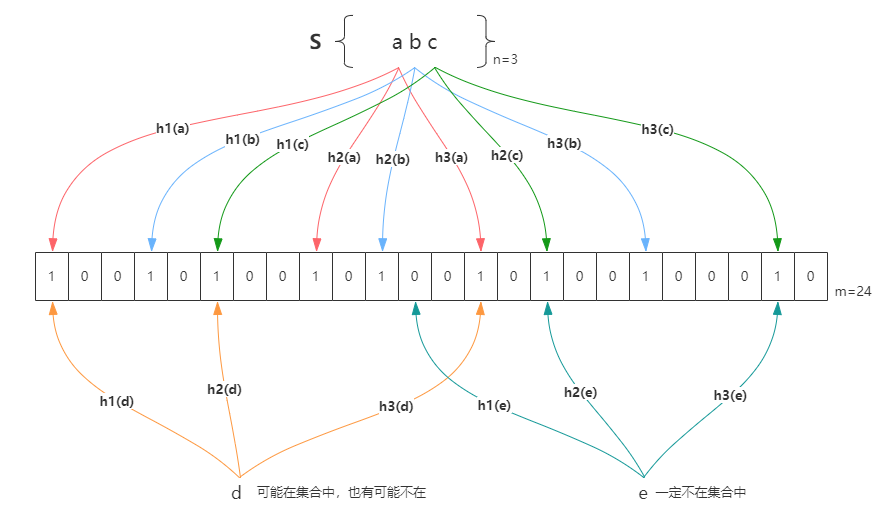# 爱吃咖喱棒的打字员DA☆ZE~

0%# 布隆过滤器参数的确定

k 次哈希运算后，某一 bit 位没有被置为 1 的概率为 $(1 - \frac{1}{m})^k$

$m = - \frac{nlnp}{(ln2)^2}$

$k = ln2\frac{m}{n}$

Bloom filter